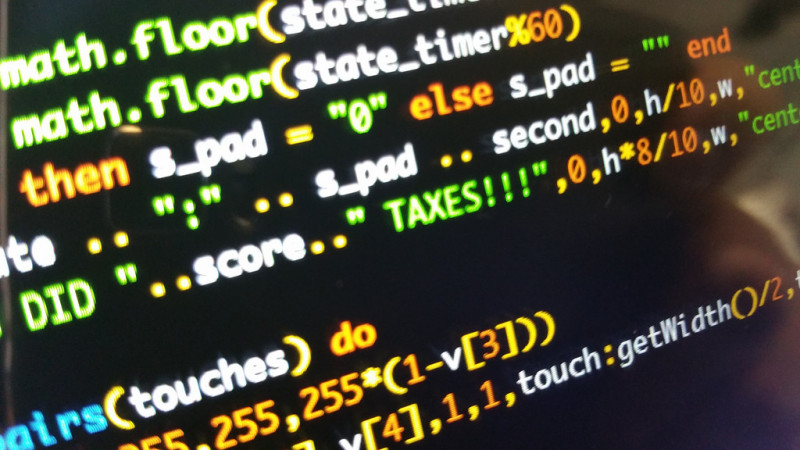# Programming improve math

2018-05-30It is an undeniable fact that programming results in many benefits, including the development of critical thinking skills, the ability to seek alternative solutions to a problem, an understanding of cause and effect, and strong planning skills. Those are just some of the indirect benefits that will be developed as you improve your programming skills.

When it comes to direct learning outcomes, the results are program-dependent. If you are learning to program in a way that focuses on science, you will of course learn about different sciences. The main and most common learning outcome in all programming languages is mathematics. Programming relies upon the development of strong math skills like cryptography, which is a mathematical science built on the use of prime numbers. Cryptography education is like solving puzzles, but it relies upon code. Needless to say, cryptography is an important element of computer programming.

Computers use binary data, which is a numeric system that uses only the digits 0 and 1. The electronic components used to build computers only recognize two different states, on, which is represented by a 1, and off, which is represented by a 0. Hexadecimal, another common numeric system used by computers, has six more digits than our standard 0-9 numeric system can provide. This is shown by using the letters A through F to represent the digits above 9.

You don’t need math to write your first “Hello World” program, but when you’re trying to demonstrate why your program is the best option you will need math to prove your point. There is an advantage to knowing certain mathematical concepts before jumping into the field of programing. You will need to understand simple mathematical operations like addition, subtraction, multiplication, division, and mod, which is the remainder of a division operation. Decimal point operations like ceil and floor, which approximate a decimal number to the nearest upper or lower integer number, can come in handy as well. Finally, it is important to understand basic numbering formats, including decimal, binary, hexadecimal, and octal numbers.

In conclusion, mathematics is programming and vice-versa. For children to learn how to code, they must know and understand the basics of math. When instructors try to teach children who lack a strong mathematical background how to code, they are disappointed by the number of math-related questions they receive. Thinking about the flow of a programming problem exercises the same mental muscles that are used to think of a geometry or statistics problem. This unique method of thinking and problem solving is definitely worth learning.

## RELATED BLOGS

#### RoboGarden

Do you want to learn coding in less time than possible and without any money, join us on the best platform ever on learning coding as a game for kids?

#### Importance of coding educationPrivacy Policy Terms of Use